# Iterative Binary Tree Traversal using Stack: Preorder, Inorder and Postorder

### Introduction to iterative tree traversals

In all recursive DFS traversals of a binary tree, we have three basic elements to traverse— root, left subtree, and right subtree. Each traversal process nodes in a different order using recursion, where recursive code is simple and easy to visualize i.e. one function parameter and 3–4 lines of code. So critical question would be — how do we convert it into iterative code using stack? Let’s think.

Stack is a useful data structure to convert a recursive code into an iterative code. In recursive code, under the hood in, the compiler uses a call stack to convert it into an iterative code. This call stack stores information like local variables, input parameters, the current state of the function call, etc. Sometimes iterative implementation using stack becomes complex due to many input variables, additional operations, and the complex nature of recursive calls. But in some situations, recursion is simple and we can easily convert it into iterative code. One idea works best: we need to mimic what the compiler does when we run recursive code! So the stack is the best data structure to track the execution of recursive calls.

But before moving forward to iterative implementation, let's understand the nature of recursion in DFS traversals. Both preorder and inorder traversals are tail-recursive i.e. there is no extra code operation after the final recursive call. So implementation using stack is simple and easy to understand. But postorder traversal is non-tail recursive because there is an extra operation after the last recursive calls i.e. we process the root node. So an implementation of postorder using a stack would be a little tricky. But if we follow the basic order of processing nodes, it can be easy to visualize.

To simulate the recursive traversal into an iterative traversal, we need to understand the flow of recursive calls in DFS traversal. We visit each node three times:

• First time: when recursion visits the node coming from the top. In preorder traversal, we process the node at this stage.
• Second time: when recursion backtrack from the left child after visiting all the nodes in the left subtree. In inorder traversal, we process the node at this stage.
• Third time: when recursion backtrack from the right child after visiting all the nodes in the right subtree. In postorder traversal, we process the node at this stage.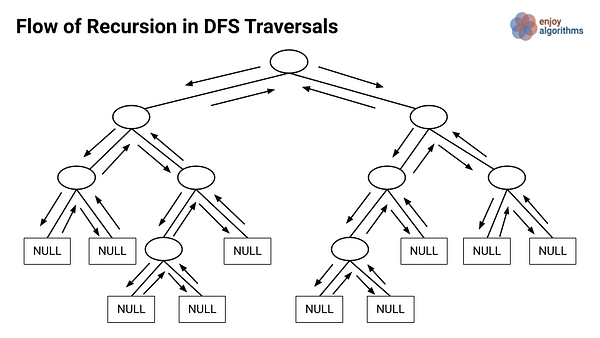Let's simulate the iterative implementation of each traversal using a stack.

### Iterative preorder traversal using stack

Let's revise the preorder traversal: we first process the root node, then traverse the left subtree, and finally, we traverse the right subtree. Tracking the flow of recursion will give a better picture.

• We first process the root. Then go to the left subtree and process the root of the left subtree. Then we continue going left in the same way until we reach the leftmost node of the tree.
• If the leftmost node is a node with only the right child: Recursion goes to the right child of the node, processes all the nodes in the right subtree using the same process, and backtrack to the node. Now traversal of the subtree rooted at the leftmost node is done and recursion backtrack to the parent node. A similar process continues for the parent node.
• If the leftmost node is a leaf node: then the traversal of the leftmost node is done and recursion backtrack to its parent node. Now it has already processed the parent node when it was coming from the top. So recursion goes to the right child of the parent node, process all the nodes in the right subtree and backtrack to the parent node. This process continues further in a similar way.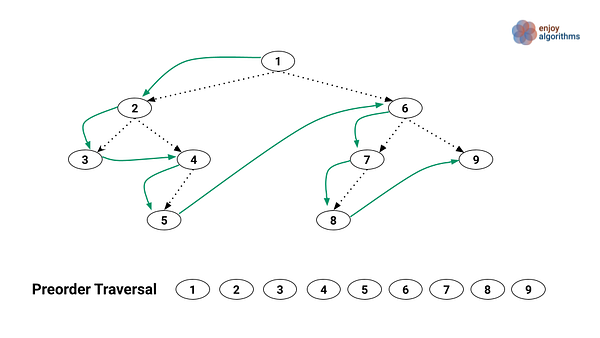Here is an idea of iterative simulation using stack: when we first time visit any node, we process it, push the node into the stack (to process the right subtree of that node later), and go to the left child. If there’s no left child, we grab one node from the top of the stack and go to the right child of that node. Now we continue the same process for the subtree rooted at the right child of the popped node. Let's understand it via the clear implementation steps.

Implantation of iterative preorder traversal

• We use a stack treeStack and a temporary pointer currNode to track the current node. At the start, we initialize currNode with the root node.
• Now we run a loop till treeStack is not empty or currNode !=NULL

Condition 1: If (currNode != NULL)

We process the currNode and push it into the treeStack. Now we move to the left child i.e. currNode = currNode->left. This process continues in a loop till we reach the leftmost end of the binary tree i.e. currNode ==Null. At this stage, all the ancestors of that node will be available on the treeStack.

``````if(currNode != NULL)
{
process(currNode)
treeStack.push(currNode)
currNode = currNode->left
}
``````

Condition 2: If (currNode == NULL)

Now we reached the leftmost end of the tree. We pop a node from the top of the treeStack and assign currNode with the right child of the popped node.

``````if(currNode == NULL)
{
prevNode = treeStack.pop()
currNode = prevNode->right
}
``````

Pseudocode of the iterative preorder traversal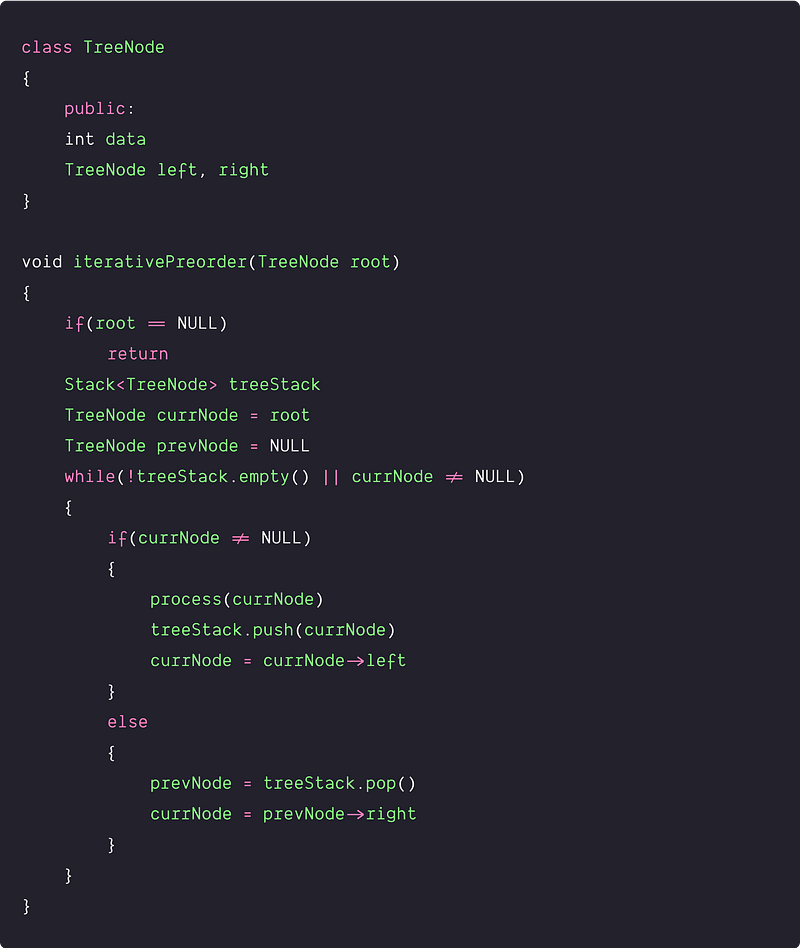### Another iterative approach of preorder using stack

If we simplify the preorder traversal for each node, we process the node first and then we process the left and right children. To track this order using stack, we push the right child before the left child to ensure that the left child is processed first. In other words, if we pop the stack, the left child comes before the right child.

Implementation steps

1. Create an empty stack treeStack and push the root node. We also use a pointer currNode to track the current node.
2. Now run a loop till treeStack is not empty.
3. Pop the top node of the stack and assign it with currNode. Now we process the currNode.
4. if(currNode->right != NULL), then we push the right child of the currNode. Otherwise, we move to the left child.
5. if(currNode->left != NULL), then we push the left child of the currNode. Now we move to the next iteration of the loop.

Pseudocode of the Implementation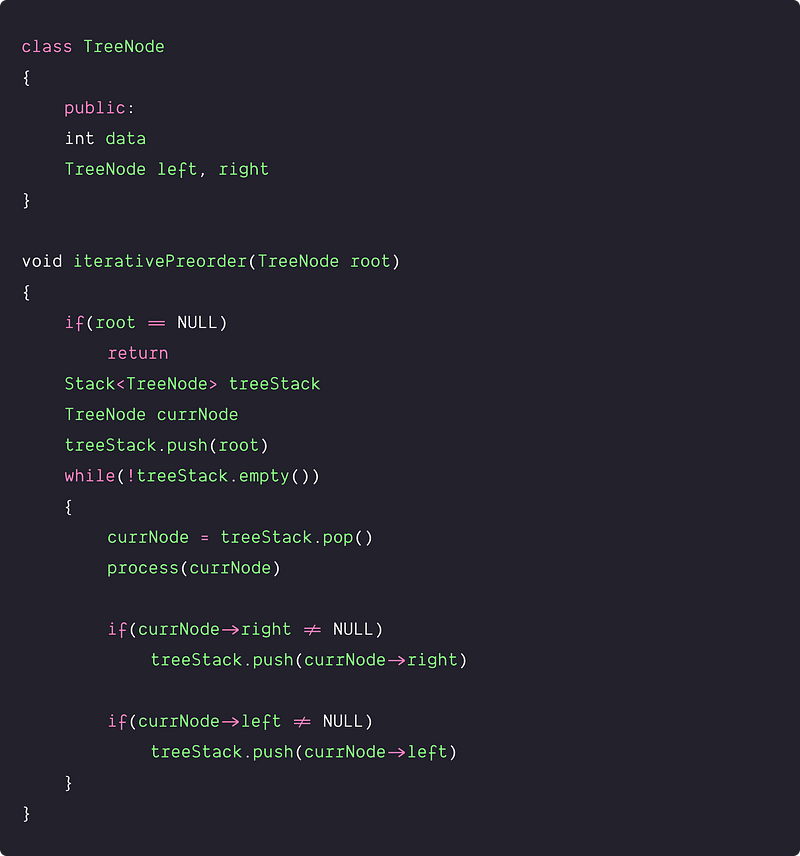Time and space complexity analysis

Each node is pushed into and popped out of the treeStack only once. So we are doing a constant number of operations for each node. Time complexity = n* O(1) = O(n).

Space Complexity: We are using one stack and the size of the stack depends on the height of the binary tree. Think! So the space complexity = O(h).

### Iterative inorder traversal  using stack

In inorder, we first process the left subtree, then process the root node, and finally process the right subtree. How do we simulate this process using a stack? Let’s understand the flow of recursion.

• We first start from the root and go to the left subtree, then go to the root of the left subtree, and so on...we reach the leftmost end of the tree. In such a way, recursion first process the leftmost node.
• If the leftmost node is a node with the right child: Recursion goes to the right child of the node, After processing all the nodes in the right subtree using the same process, recursion backtrack to the node. Now traversal of the leftmost node is done and recursion backtrack to its parent node. A similar process continues for the parent node.
• If the leftmost node is a leaf: then the traversal of the leftmost node is done and recursion backtrack to its parent node. Now it first processes the parent node, goes to the right subtree of the parent node, process all the nodes in the right subtree and backtrack to the parent node. This process continues further in a similar way.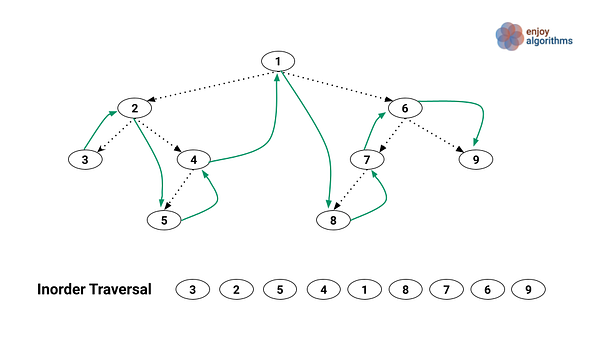Here is an idea of iterative simulation using stack: before processing the left subtree of any node, we need to save the node on a stack (to process the node and right subtree of that node). Then we go to the left child of that node. After processing all the nodes in the left subtree, we pop the node from top of the stack, process it, and go to the right child of the node to traverse the right subtree. Let's understand it via the clear implementation steps.

Implantation Steps — Iterative Inorder using stack

• Create an empty stack treeStack and push the root node. We also declare a pointer currNode to track the current node during the traversal.
• Now we run a loop till treeStack is not empty or currNode != NULL

Condition 1: If (currNode != NULL)

We push currNode into the stack for processing currNode and right subtree later. Now we move to the left child i.e. currNode = currNode->left. This step will continue in a loop till we reach the leftmost end of the binary tree i.e. currNode ==Null.

``````if (currNode != NULL)
{
treeStack.push(currNode)
currNode = currNode->left
}
``````

Condition 2: If (currNode == NULL)

Now we pop the top node from the stack, assign it with the currNode, and process it. Now we move to the right child of the currNode to traverse the right subtree of the currNode in a similar way.

``````if (currNode == NULL)
{
currNode = treeStack.pop()
process(currNode)
currNode = currNode->right
}
``````

Pseudo code of the iterative inorder traversal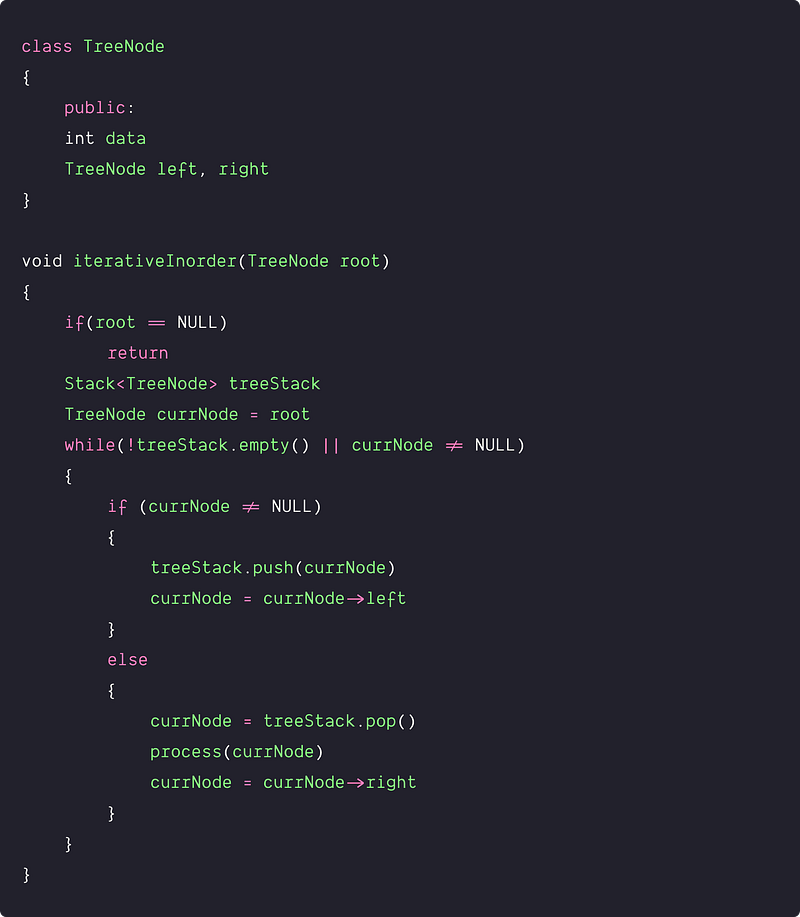Time and Space complexity analysis

Each node is pushed into and popped out of the treeStack only once. So we are doing a constant number of operations for each node. Time complexity = n* O(1) = O(n).

Space Complexity: We are using one stack and the size of the stack depends on the height of the binary tree. Think! So the space complexity = O(h).

### Iterative postorder traversal  using stack

In the postorder, we first process the left subtree, then we process the right subtree, and finally process the root node. In other words, before processing any node, we process all the nodes in the left and right subtree. How do we simulate this process using a stack? First, let’s track the flow of recursion.

We start from the root and go to the left subtree, then we go to the root of the left subtree, so on we reach the leftmost end of the tree. From here, recursion backtracks to its parent node and goes to the right child. Then, after processing all the nodes in the right subtree using the same process, recursion backtrack to the parent node and process it.

• We first start from the root and go to the left subtree, then go to the root of the left subtree, and so on...we reach the leftmost end of the tree.
• If the leftmost node is a node with the right child: Recursion goes to the right child of the node. After processing all the nodes in the right subtree, recursion backtrack to the node and process it. Now traversal of the leftmost node is done and recursion backtrack to its parent node. From here, recursion goes to the right child of the parent node, process all the nodes in the right subtree, backtrack and finally process the parent node. This process continues in a similar way.
• If the leftmost node is a leaf: the recursion process the leftmost node and traversal of the leftmost node is done. Now recursion backtrack to its parent node. From here, recursion goes to the right child, process all the nodes in the right subtree of the parent node, backtrack to the parent node, and finally process the parent node. This process continues in a similar way.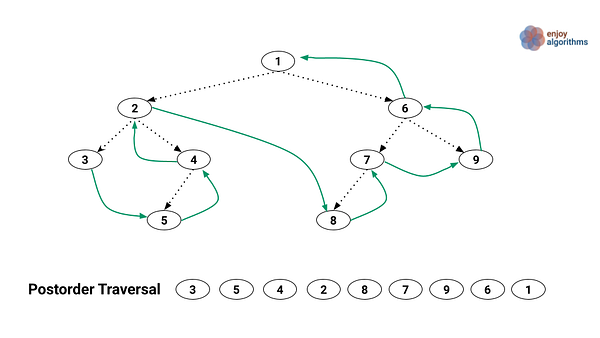Here is an idea of iterative simulation using stack: Before processing the left subtree of any node, we need to save two things on the stack: 1) Right child of the current node so that we can process right subtree after the traversal of left subtree 2) Current node, so that we can process it after the traversal of right subtree. To simulate this process, we can use two separate stacks. But how do we track the nodes in a postorder fashion? Let’s understand it clearly via the implementation.

Implantation steps : Iterative postorder using two stacks

• We declare two separate stacks: rightChildStack to store the right child and mainStack to store the current node. We also initialize an extra pointer currNode to track the current node during the traversal.
• Now we run a loop till mainStack is not empty or currNode != NULL.

Condition 1: If (currNode != NULL)

If the right child of the currNode is not NULL then push the right child into the rightChildStack. Now we push the currNode into the mainStack and go to the left child. We continue the same process until we reach the leftmost end of the tree i.e currNode ==NULL.

``````if(currNode != NULL)
{
if(currNode->right != NULL)
rightChildStack.push(currNode->right)
mainStack.push(currNode)
currNode = currNode->left
}
``````

Condition 2: If (currNode == NULL)

Now we access the parent node of the currNode which is present at the top of the mainStack. We access the top node from the mainStack and assign it with currNode. But before processing the currNode, we need to process nodes in the right subtree.

• If the rightChildStack is not empty and the top node of the rightChildStack is equal to the right child of the currNode, it means, we have not yet traversed the subtree rooted at the right child, so we pop the top node from the rightChildStack and assign it with currNode.
• Otherwise, traversal of the right subtree of the currNode is done, and we finally process the currNode. Now we pop the top node from the mainStack and assign the currNode equal to the NULL (Think). In this case, the loop again goes to condition 2 and repeats the same steps until currNode is not equal to NULL.
``````currNode = mainStack.top()
if(!rightChildStack.isEmpty() && currNode->right == rightChildStack.top())
currNode = rightChildStack.pop()
else
{
process(currNode)
mainStack.pop()
currNode = NULL
}
``````

Pseudocode of the iterative postorder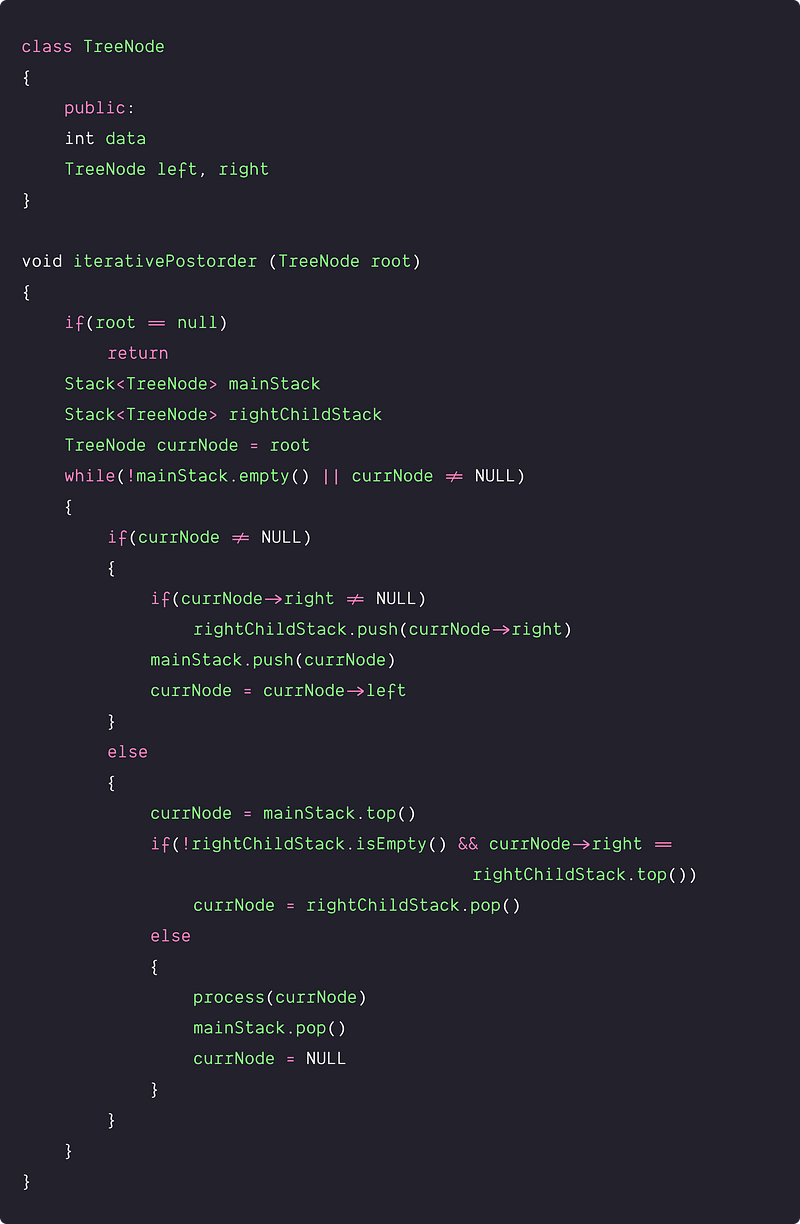Time and space complexity analysis

Each node is pushed into and popped out of the mainStack only once. Similarly, in the worst case, each node is pushed into the rightChildStack at most once. So we are doing a constant number of operations for each node. Time complexity = n* O(1) = O(n).

Space Complexity: We are using two different stacks where the size of each stack depends on the height of the binary tree. Think! So the space complexity = O(h).

### Critical ideas to think!

• Can we optimize the postorder traversal using two stacks? Is there any way to implement the post-order traversal using a single stack? Here is an implementation code using the one stack and O(n) extra memory. Is it look similar to the two-stack implementation? Think!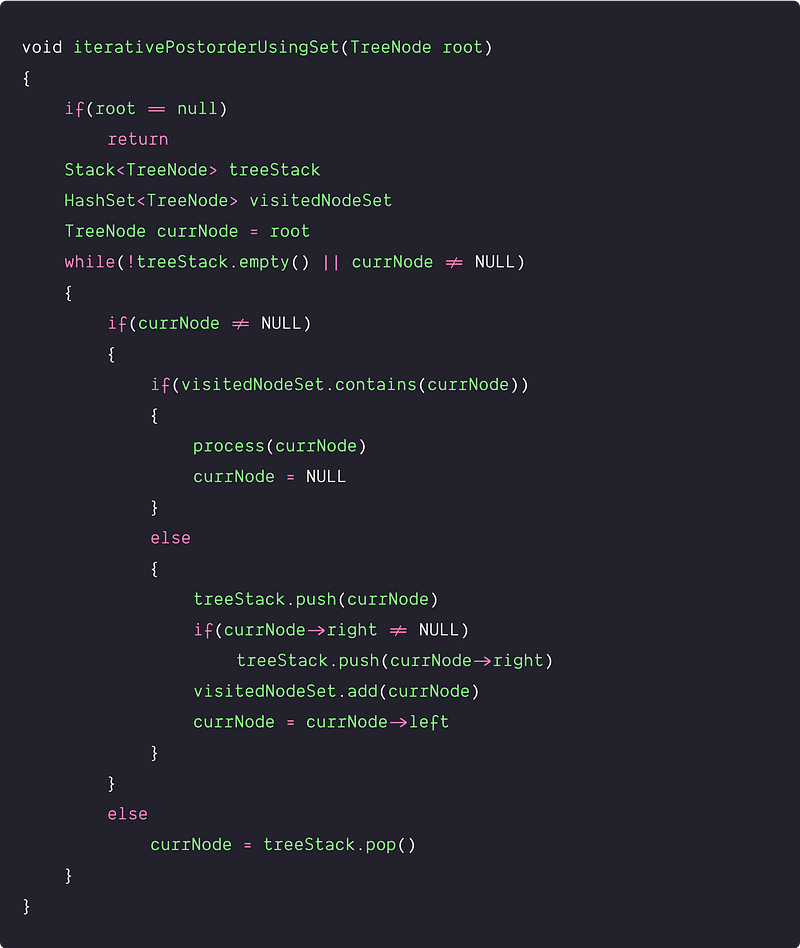• How do we implement all the iterative DFS traversals without using stack? Idea: Threaded binary tree traversal or Morris Traversal.
• What would be the worst, best and average case of the space complexity?
• In the postorder traversal, how many operations will be performed on rightChildStack in the best and worst case?

### Critical concepts to explore further!

• Recursive DFS traversal using stack
• BFS Traversal of binary tree
• BFS vs DFS traversal comparison
• Properties and Types of binary tree
• Various binary tree operations like insertion, deletion, construction, height, merging, searching, and other transformation and query operations.
• Array-based implementation of the binary tree
• Properties and operations of Binary Search Tree

### Problems to solve using DFS traversals

• Left View of Binary Tree
• Maximum width of a binary tree
• Min Depth of Binary Tree
• Level with a maximum number of nodes
• Convert a binary tree into a mirror tree
• Find the diameter of the binary tree
• Print nodes at k distance from a given node
• Boundary Traversal of the binary Tree
• Least Common Ancestor of two nodes in Binary tree
• Construct binary tree from preorder and In-order Traversal

Enjoy learning, Enjoy coding, Enjoy algorithms!

Get well-designed application and interview centirc content on ds-algorithms, machine learning, system design and oops. Content will be delivered weekly.